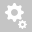##Recently viewed ticketsQuestion:

why is the moment diagram shown by midas is wrong/opposite since the lateral load is not suction?Hi,

There is an option called 'Reverse' with which you can control the direction of moment diagram as shown below.Regards,
DK Lee

so do you mean that the different here is only the axis of the plate element?

because if you are going to look at the floor moment diagram with gravity loads, the bending moment seems to be similar with the deformation momenthow come that the wall moment diagram seems different?

Hi,

In the 'Plate Cutting Line Diagram' results, the moments are calculated with respect to the axes which are determined from the three points, i.e. P0, P1 and P2 as shown below. The x axis is determined from P0-P1 vector. The y axis is perpendicular to the x axis and the positive direction of the y axis is determined based on the location of P2. The z axis is normal to the plane which is defined with P0, P1 and P2.
Therefore, the direction of moment diagram or the sign of moments (positive or negative) is determined based on the location of P0, P1 and P2. The local axes of plate elements do not affect the results.Regards,
DK
Creation date: 8/15/2018 8:25 PM (dklee@midasit.com)      Updated: 8/16/2018 5:56 AM (dklee@midasit.com)